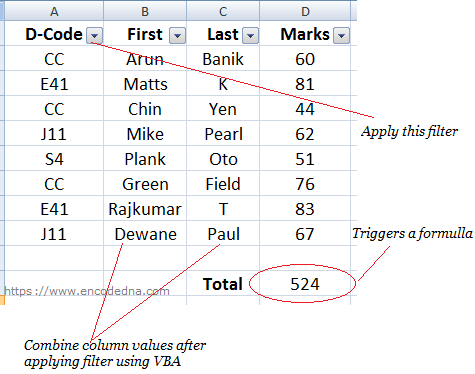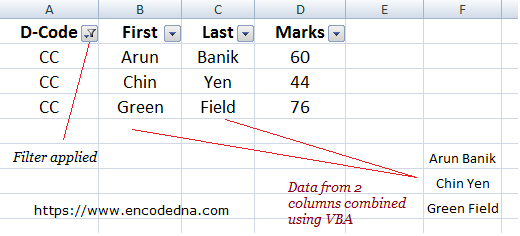﻿ Capture Filter Change Event in Excel using VBA or how to Trigger a Macro Function on Filter Change

# Capture Filter Change Event in Excel using VBA or how to Trigger a Macro Function on Filter Change

A filter in Excel, as you know, helps in filtering a range of data in a worksheet. It helps in narrowing down information by hiding unnecessary data, temporarily. You can apply filter to all the columns or a single column as per your choice. Now, here is a query (I have recently answered in a forum) on how to capture filter change event using VBA.

## A Simple Scenario

I have four columns of data (see the below image) in my worksheet. The second and third columns have first name and last name, respectively. Now, when someone filters the range of data (using filter in first column), I wish to capture the filter change event and pick up the values of second and third column, combine it and finally show it. I wish to combine both the names.Now here’s the trick. I’ll add a formula to my worksheet. I can apply the Sum() function at the end of the fourth (Marks) column. Whenever I filter the data, it will trigger the formula, which in turn will fire an event called Worksheet_Calculate().

Note: You can actually add a dummy formula (anywhere in the worksheet) and that too will fire the event. For example, I’ll add the below formula in my worksheet and it will point to A1 cell.

=A1

It does nothing but shows the value inside the cell A1. However, I want to add a real formula, therefore I have the Sum() function.

I’ll write the VBA event WorkSheet_Calculate() in the Sheet1 module, since the data that I am filtering is in sheet1.

The VBA Code
```Option Explicit

Private Sub Worksheet_Calculate()
If ActiveSheet.Name = "Sheet1" Then
If Cells(Rows.Count, 1).End(xlUp).Row > 1 Then

Dim iRow As Integer
iRow = 15           ' THE ROW TO SHOW FILTERED DATA.

' CLEAR THE COLUMN WITH PREVIOUS DATA (IF ANY).
Sheet1.Columns(6).ClearContents

' THE RANGE OF DATA THAT IS VISIBLE AFTER APPLING THE FILTER.
Dim MyRange As Range
Set MyRange = ActiveSheet.Range("B2:B10").Rows.SpecialCells(xlCellTypeVisible)

Dim rowCell As Range
For Each rowCell In MyRange.Cells
Sheet1.Cells(iRow, 6) = rowCell.Cells(1, 1) & " " & rowCell.Cells(1, 2)
iRow = iRow + 1
Next rowCell
End If
End If
End Sub```

### OutputWell that’s it. Hope the above example is useful. Thanks for reading.